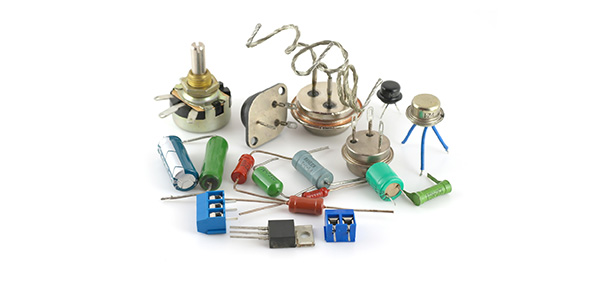6 Questions | Total Attempts: 102SettingsExample questions focusing on brady pacing.

Related Topics
• 1.
Voltage is...
• A.

The electromotive force pushing on electrons

• B.

Moving electrons

• C.

The opposition to current flow

• D.

Current divided by resistance

• 2.
If the Pacemaker output voltage is 5v and the measured lead resistance is 330 ohms, then the  current that flows out of the pacemaker into the heart is:
• A.

1.65 mA

• B.

15.15 mA

• C.

66 mA

• D.

10 mA

• 3.
If a unipolar lead wire has an insulation break one would expect the resistance to:
• A.

Stay the same

• B.

Rise

• C.

Decrease

• D.

None of the above

• 4.
For a wire fracture the resistance:
• A.

Increases

• B.

Decreases

• C.

Increases then decreases

• D.

Has no change

• 5.
If the output voltage of the pacemaker is programmed from 5 volts to 2.5 volts, the energy  delivered to the heart is:
• A.

Doubled

• B.

Halved

• C.

Quartered

• D.

• 6.
Which of the following affect the longevity of the pacemaker?
• A.

Output Voltage

• B.